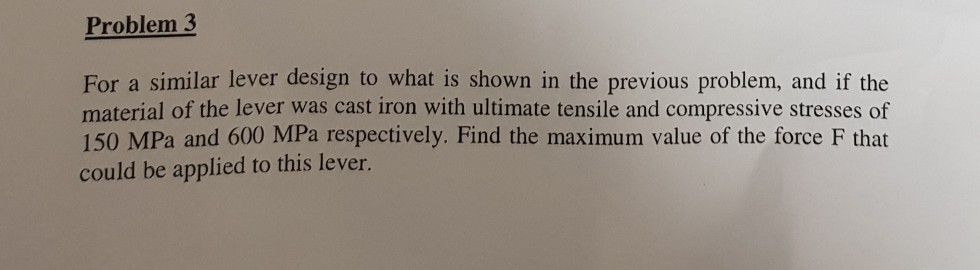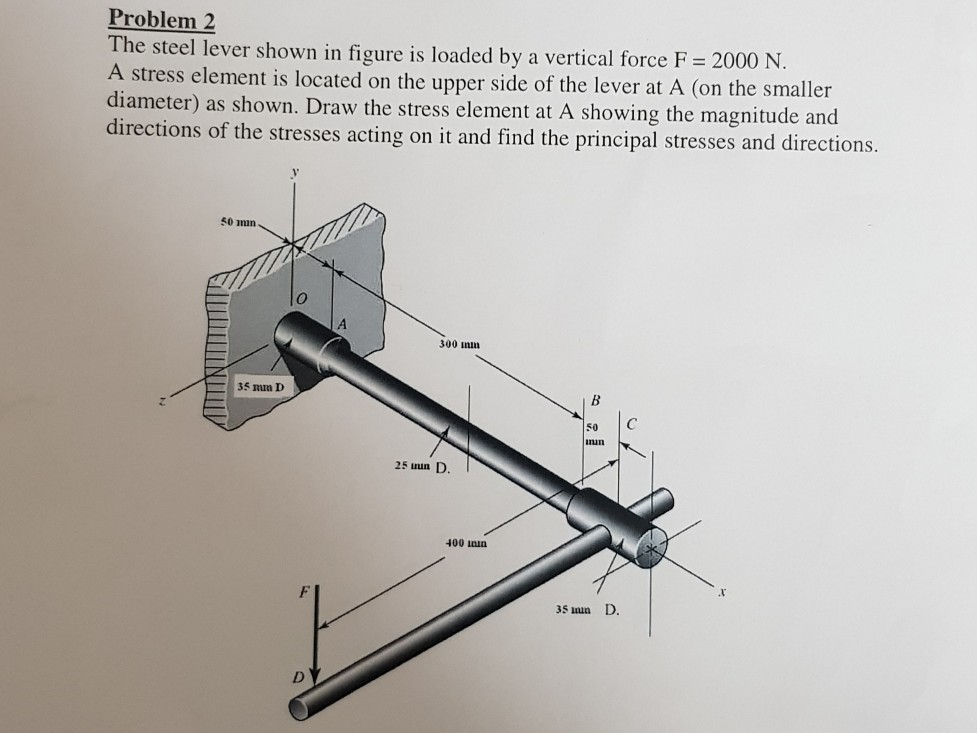# Question Problem 2 The steel lever shown in figure is loaded by a vertical force F= 2000 N. A stress element is located on the upper side of the lever at A (on the smaller diameter) as shown. Draw the stress element at A showing the magnitude and directions of the stresses acting on it and find the principal stresses and directions. 50 min 300 mm 35 mm D B SO un 25 in D 400 min 35 un D. DR9ECYF The Asker · Mechanical EngineeringTranscribed Image Text: Problem 2 The steel lever shown in figure is loaded by a vertical force F= 2000 N. A stress element is located on the upper side of the lever at A (on the smaller diameter) as shown. Draw the stress element at A showing the magnitude and directions of the stresses acting on it and find the principal stresses and directions. 50 min 300 mm 35 mm D B SO un 25 in D 400 min 35 un D. D
More
Transcribed Image Text: Problem 2 The steel lever shown in figure is loaded by a vertical force F= 2000 N. A stress element is located on the upper side of the lever at A (on the smaller diameter) as shown. Draw the stress element at A showing the magnitude and directions of the stresses acting on it and find the principal stresses and directions. 50 min 300 mm 35 mm D B SO un 25 in D 400 min 35 un D. D